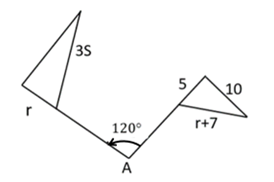Mathematics
Easy

Question

# What is the value of ‘s’ in the rotation of the triangle about A?## 3    4    5    6Hint:

## The correct answer is: 4

### Rotation is isometry.r = 53s = r + 7 (substitute r = 5)3s = 5 + 73s = 12s = 4.

corresponding parts of triangles remain same so equate them and get the Unknown values.

### Related Questions to study#### With Turito Foundation.#### Get an Expert Advice From Turito.